So, Gibbs sampling is one way (among many) to estimate a Bayesian model. It’s also one of the many estimating techniques contained under the hood of MLWiN. If you’re really interested, I’d highly recommend Marin & Robert’s Bayesian Essentials with R (I have a copy I don’t use if the library doesn’t have it). It’s a great, simple introduction to the relevant concepts (and importantly, makes you actually apply them).

So, you’re probably familiar with things like maximum likelihood estimation, of which ordinary least squares estimators are a type. Usually, these methods focus on the likelihood function, which is an inversion of the probability problem of concluding the probability of your theory $$\theta$$ (usually some parameter of interest about a process) from some data $$y$$.

$p(\theta | y) \propto \mathcal{L}(y | \theta)$ where $$\mathcal{L}$$ is a likelihood function. Likelihoods are not probabilities, but they’re related to them.

Usually, Bayesians interpret this statement as saying the probability of your belief given the data should be proportional to the probability of the data given your belief.

$p(\text{belief | data}) \propto \mathcal{L}(\text{data | belief})$

But, this is actually missing one part. Formally, this likelihood relationship actually comes from Bayes’ Theorem. Since we observe $$y$$, the data, and want to get the probability our theory $$\theta$$ is true, we use Bayes’ Theorem: $p(\theta | y) = \frac{p(y | \theta) p(\theta)}{p(y)}$ Now, for estimation purposes, we drop $$p(y)$$ because it’s constant with respect to $$\theta$$: if $$y$$ is “known,” the probability we would observe it over infinite replications is fixed. This means we’re left with

$p(\theta | y) \propto p(y | \theta) p(\theta)$

Or, in the plain language above, the probability of your belief given the data should be proportional to the probability of the data given your belief, adjusted by the probability of your beliefs are true. $p(\theta | y) \propto p(y | \theta) p(\theta)$ or $p(\text{belief | data}) \propto \mathcal{L}(\text{data | belief}) p(\text{belief before observing data})$

We call $$p(\theta)$$ a “prior probability,” and you might’ve worked with these already in MLWiN.

So, say we have a simple linear regression $$y = X\beta + \epsilon$$: \begin{align} y &= X\beta + \epsilon \\ \epsilon \sim \mathcal{N}(0,\sigma^2) \end{align} Now, to complete the model, we assign the parameters $$\beta,\sigma$$ a prior. For Gibbs sampling, we need to pick special priors to make the problem tractable. We’ll let $$\beta ~ \mathcal{N}(\mu, \tau^2)$$, where $$\mu$$ is our “prior belief” about the average $$\beta$$ effect we might observe, and $$\tau^2$$ is how intrinsically noisy we think the effect is. For $$\sigma^2$$, our prior parameters will correspond to how many observations we think our prior belief about the model variance should be “worth” relative to the observations, and the magnitude of intrinsic noise in the data. \begin{align} \beta &\sim \mathcal{N}(\mu, \sigma^2) \\ \beta &\sim \text{Inverse Gamma}(a,b) \end{align} This is only one possible specification. The inverse gamma distribution is commonly used for modeling the uncertainty around unknown variances, and assigning the $$\beta$$ parameters a normal prior where $$\mu = 0$$ is also a common choice. Regardless, since $$\epsilon$$ is normally distributed, our model of $$y$$ conditional on our unknown parameters is the typical normal distribution used in regression: $y | (\beta, \sigma) \sim \mathcal{N}(X\beta, \sigma^2)$ This works because, if $$\beta$$ was known, the mean of $$y$$ is simply $$X\beta$$. Our error is modeled as normally distributed with mean 0 and variance $$\sigma^2$$ by the Gauss-Markov theorem.

Pulling this all back together with the information about $$p(\theta)$$, our priors, we can use that statement about belief & data we made before to structure our belief after having observed data (sometimes called the “posterior belief” because it’s post-observation): $p(\beta, \sigma^2) \propto p(y | \beta, \sigma^2) p(\beta) p(\sigma^2)$ This is pretty complex, and is a function of two parameters, $$\beta, \sigma$$. But, we know the distributional forms for each of the components on the right hand side: $p(\beta, \sigma^2) \propto \mathcal{N}(X\beta, \sigma^2) \mathcal{N}(\mu, \tau^2) \text{IG}(a,b)$ This is pretty complex as it stands, so we’re going to try to simplify it a little more.

Gibbs sampling

Gibbs sampling reduces this statement to a sequence of distributions that are a function of a single parameter. For instance, what if we just pretended we knew what $$\sigma^2$$ was? Then, the disribution $$p(\beta | y, \sigma^2)$$ would be much simpler: $p(\beta | y, \sigma^2) \propto \mathcal{N}(X\beta, \sigma^2) \mathcal{N}(\mu, \tau^2)$ If you do some algebra, you can work out the fact that this turns into: $\beta | y, \sigma^2 \sim \mathcal{N}(m, s)$ where $$m$$ and $$s$$ are now mixtures of the prior information and information in the data. In fact, if you do the algebra, it’ll make clear that $$m$$ and $$s$$ have some familiar forms: $m = (X'X)^{-1}X'y \ \ \ \ \ \ \ \ s = \sigma^2(X'X)^{-1}$ That is, our prior for $$\beta$$ is normal, and our “conditional posterior” for $$\beta$$ is normal. This is called “conditional conjugacy,” when the distribution of one of our parameters (assuming everything else is known) is in the same form as our prior belief about the parameter. If we did know $$\sigma^2$$, we could just draw from this using rnorm in R!

This realization is what Gibbs sampling leverages.

Second, the parameters of $$\beta$$ should be recognizable from a linear regression equation. Specifically, $$m$$ is the estimator for $$\hat{\beta}$$ in a typical linear regression! But, since we’ve now incorporated some uncertainty into $$\beta$$ using on our prior belief, $$\beta$$ is considered as randomly distributed around that value. Regardless of this uncertainty, on average, $$\beta$$ will be its least squares estimate. Its randomness around the least squares estimate depends on the *empirical covariance, $$(X'X)^{-1}$$, and whatever our assumed-known value of $$\sigma^2$$ is.

For $$\sigma^2$$, we now will pretend we know $$\beta$$. This also simplifies the distribution of $$\sigma^2$$ alone: $\sigma^2 | y, \beta \sim \mathcal{N}(X\beta, \sigma^2) \text{IG}(a,b)$ This is the distribution of $$y$$ times our prior belief about $$\sigma^2$$, as was the case for $$\beta$$.

Again, if you do the algebra, this turns out to be conditionally conjugate as well: $\sigma^2 | y, \beta \sim \text{IG}(a_n,b_n)$ where $a_n = \frac{n}{2} + a \ \ \ \ \ \ \ \ b_n = (y - X\beta)'(y - X\beta) / 2$ If we only knew $$\beta$$, this could be sampled using rinvgamma from MCMCpack.

For below, we’ll let every “hyperparameter,” $$\mu, \tau^2$$ for $$\beta$$ and $$a,b$$ for $$\sigma^2$$, be small enough that we can just drop them from our expressions below. They make things slightly more complicated, and I’m trying to keep this simple. But, Chapter 3 of Bayesian Essentials with R would cover this in more detail.

The Trick

The trick with this is that we can just start at some good-enough value of $$\beta$$ or $$\sigma^2$$, draw the other one , and then use value to draw a better value of the first parameter we assumed. I’ll be using a subscript to denote that we’re in some “iteration” of the sampler. So, $$\beta_k$$ is the $$\beta$$ value we’ve constructed at the $$k$$th iteration.

Say, for example, we just arbitrarily state a first guess for $$\beta$$ is zero, so $$\beta_1 = 0$$. Then, we’d draw sigma randomly from: $\sigma^2_1 | y, \beta \sim \text{IG}(\frac{N}{2},(y - X \bullet 0)'(y - X\bullet 0)/2)$ Then, we could use this $$\sigma^2_1$$ we’ve just drawn as if it’s “known” and draw our next iteration’s $$\beta$$, called $$\beta_2$$, from: $\beta_2 | y, \sigma^2 \sim \mathcal{N}((X'X)^{-1}X'y, \sigma^2_1 (X'X)^{-1})$ Theorems demonstrated by Geman & Geman (1984) demonstrate that, if we do this back & forth for a long time, the draws of $$\beta$$ and $$\sigma^2$$ will get arbitrarily close to the “correct” distributions for $$\beta$$, $$\sigma^2$$.

The course of parameter draws taken over iterations is what you’d see as a “traceplot” in MLWiN. Usually, we only analze the end of the trace, since that’s assumed to be drawn from the “correct” distribution. But, implementing this in R is pretty straightforward.

Just for an example, let’s use the R trees dataset, and do a regression on tree volume using Girth and Height.

data(trees)
attach(trees)
library(MCMCpack)
## ##
## ## Markov Chain Monte Carlo Package (MCMCpack)
## ## Copyright (C) 2003-2018 Andrew D. Martin, Kevin M. Quinn, and Jong Hee Park
## ##
## ## Support provided by the U.S. National Science Foundation
## ## (Grants SES-0350646 and SES-0350613)
## ##
plot(Height, Volume, data=trees)
## Warning in plot.window(...): "data" is not a graphical parameter
## Warning in plot.xy(xy, type, ...): "data" is not a graphical parameter
## Warning in axis(side = side, at = at, labels = labels, ...): "data" is not
## a graphical parameter

## Warning in axis(side = side, at = at, labels = labels, ...): "data" is not
## a graphical parameter
## Warning in box(...): "data" is not a graphical parameter
## Warning in title(...): "data" is not a graphical parameter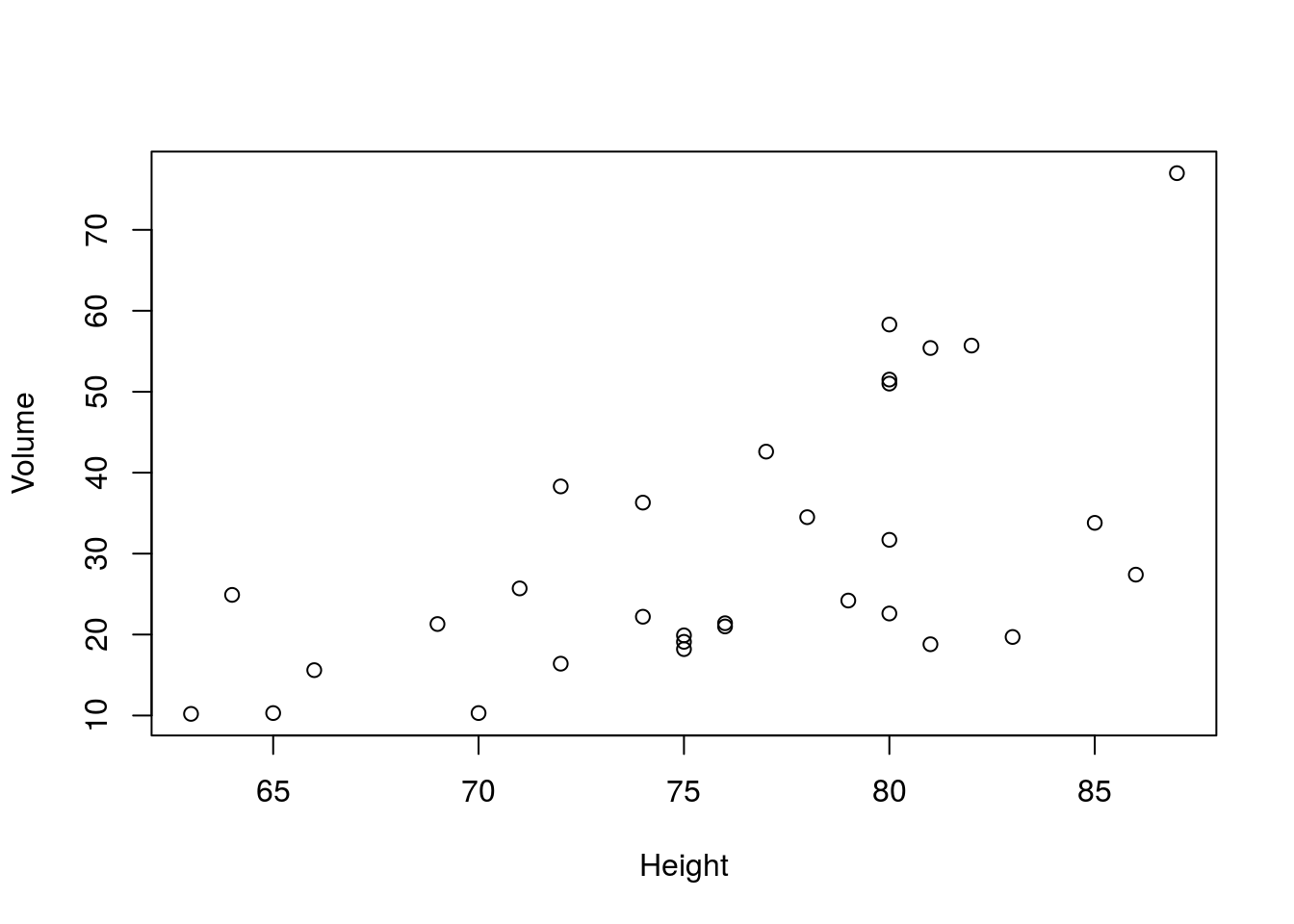plot(Girth, Volume, data=trees)
## Warning in plot.window(...): "data" is not a graphical parameter
## Warning in plot.xy(xy, type, ...): "data" is not a graphical parameter
## Warning in axis(side = side, at = at, labels = labels, ...): "data" is not
## a graphical parameter

## Warning in axis(side = side, at = at, labels = labels, ...): "data" is not
## a graphical parameter
## Warning in box(...): "data" is not a graphical parameter
## Warning in title(...): "data" is not a graphical parameter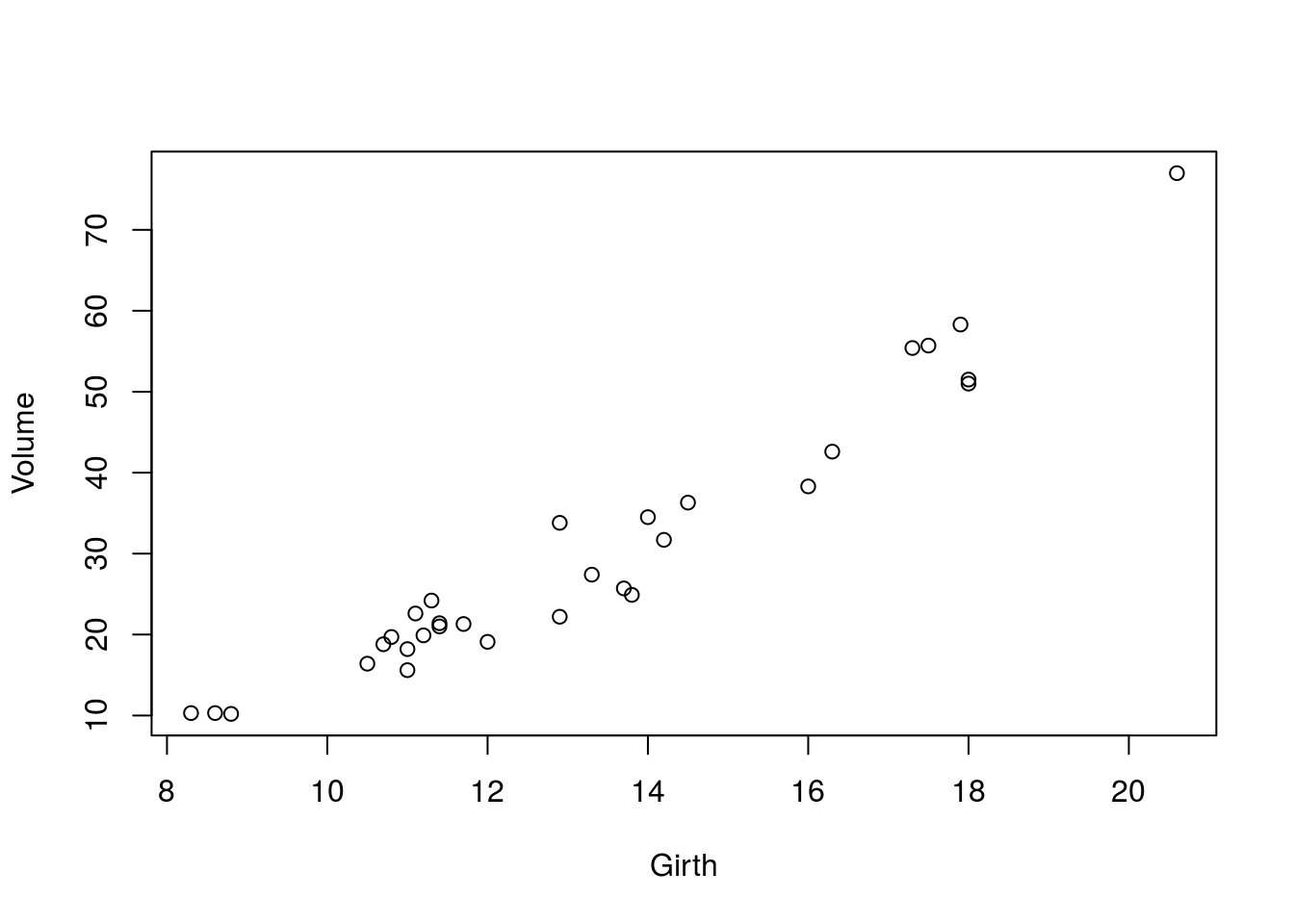X = cbind(trees$Girth, trees$Height)
y = trees$Volume n_obs = length(y) X = cbind(rep(1, n_obs), X) # gonna include a constant XtX = t(X) %*% X n_params = 3 # two covariates and a constant beta_hat = solve(XtX, t(X) %*% y) # compute this ahead of time cause we'll need it a lot XtXi = solve(XtX) beta = c(0,0,0) # starting value sigma2 = 0 #starting value n_iterations = 5000 beta_out = matrix(data=NA, nrow=n_iterations, ncol=n_params) sigma_out = matrix(data = NA, nrow = n_iterations, ncol=1) for (i in 1:n_iterations){ beta = mvrnorm(n=1, beta_hat, sigma2 * XtXi) # need the multivariate cause we have three beta part = (y - X %*% beta) sigma2 = rinvgamma(1, n_obs/2, t(part) %*% part * .5 ) beta_out[i,] = beta sigma_out[i,] = sigma2 } Now, the distribution of the coefficients in the two simulation matrices, beta_out and sigma_out, is a real sample from the distributions of the parameters. You can verify for yourself by first estimating the model using lm: linreg = lm(Volume ~ Girth + Height, data=trees) and plotting them together. Below, I’ll plot the effect estimated by lm in a red vertical line, and the full distribution of the estimates from Gibbs sampling in the histogram. For the intercept: hist(beta_out[,1]) abline(v=linreg$coefficients, col='red')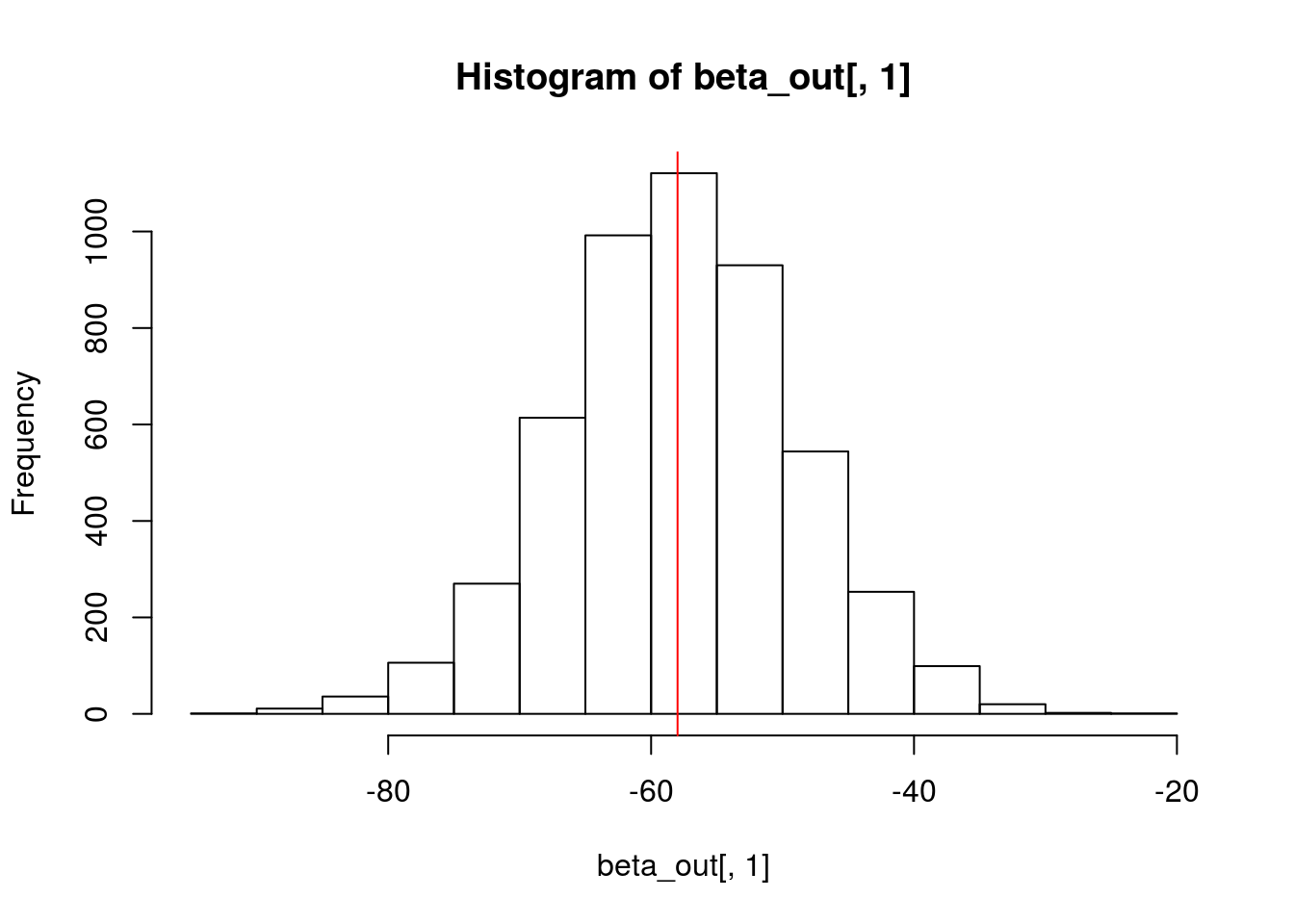The effect of girth on volume:

hist(beta_out[,2])
abline(v=linreg$coefficients, col='red')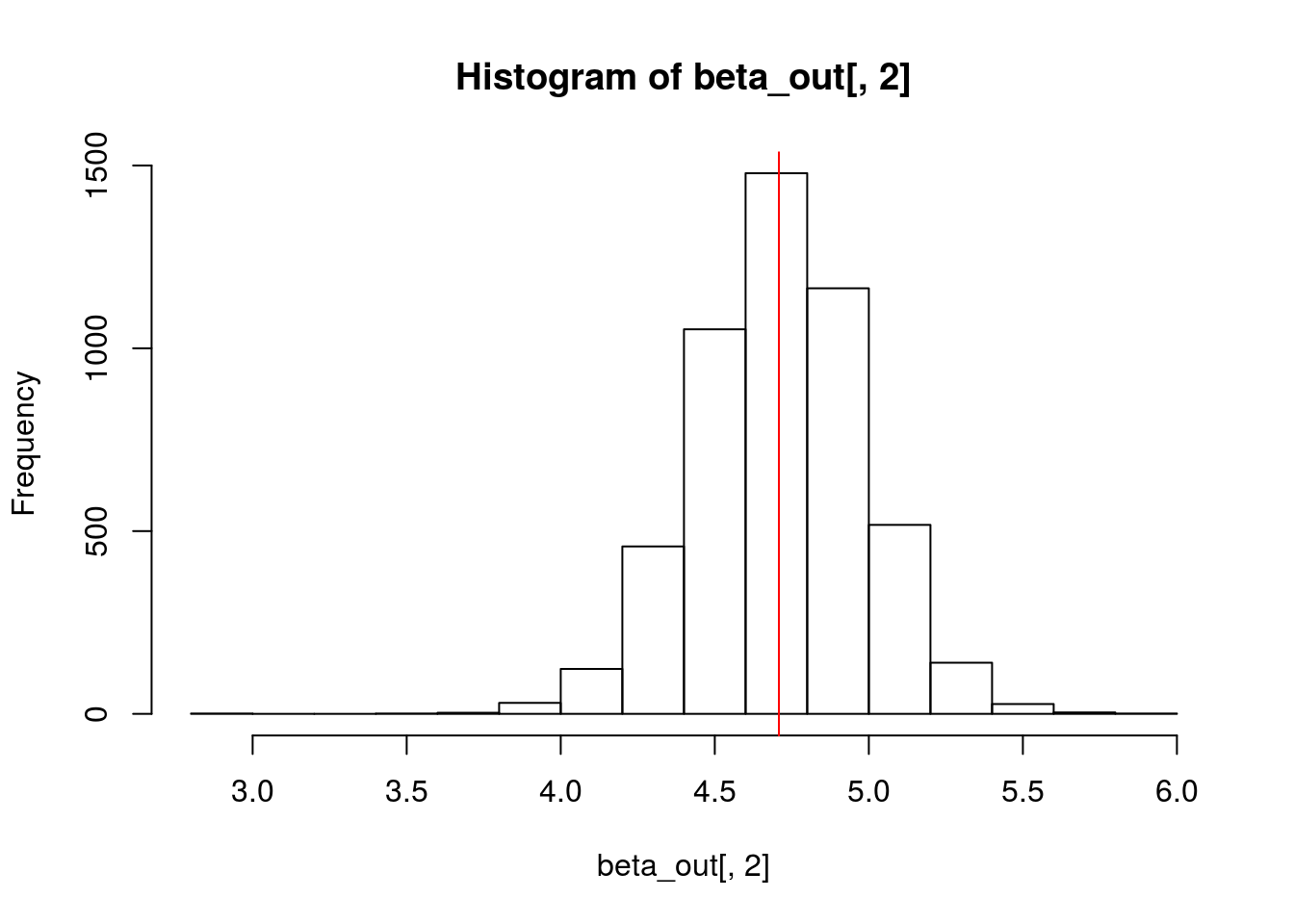and the effect of tree height on trunk volume: hist(beta_out[,3]) abline(v=linreg$coefficients, col='red')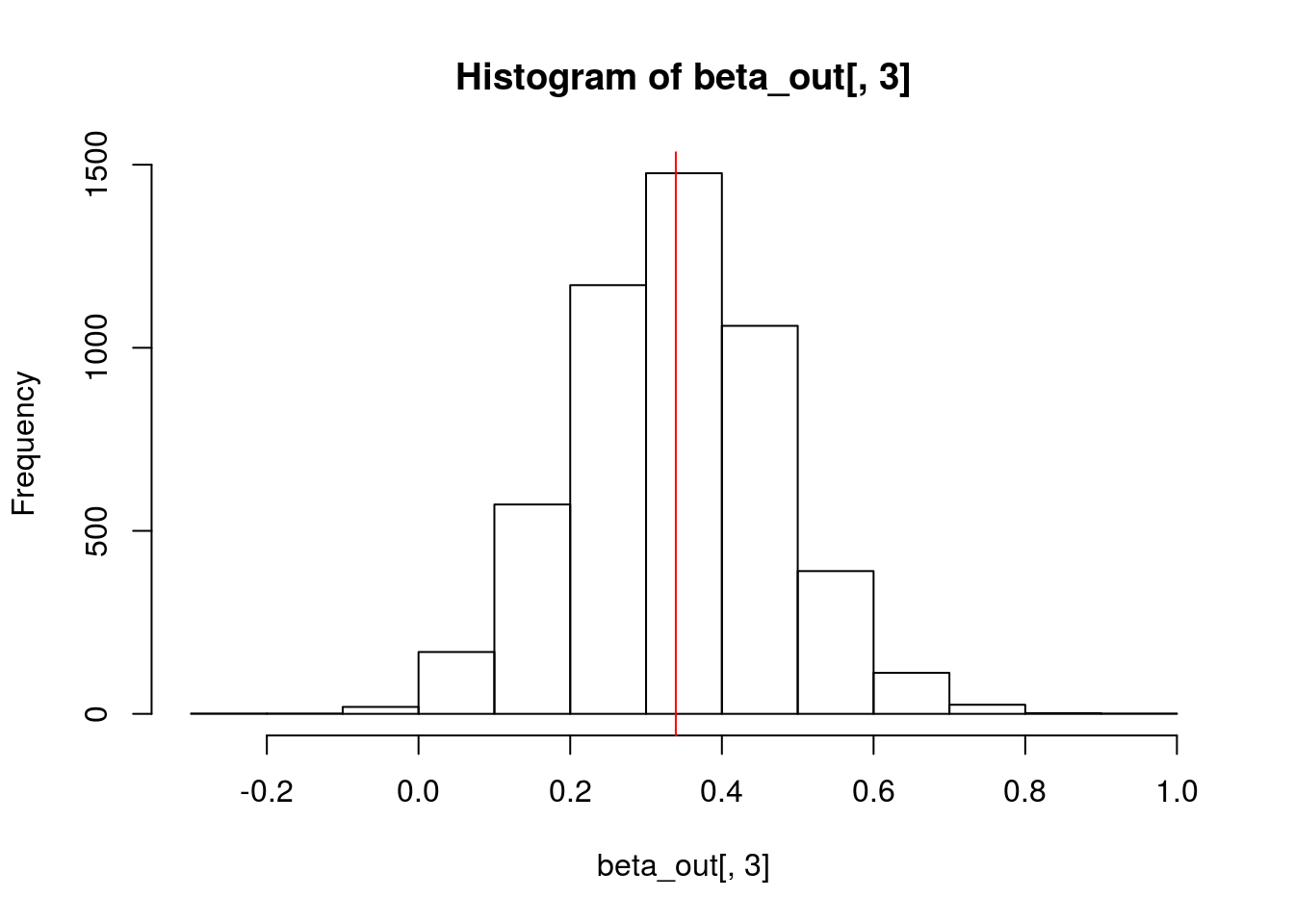Further, you can see whether the estimation is comparable in efficiency by comparing the standard deviation of the posterior samples to the standard error of the regression:

posterior_sds = apply(beta_out, 2, sd)
std_errs = sqrt(diag(vcov(linreg)))
print(cbind(posterior_sds, std_errs))
##             posterior_sds  std_errs
## (Intercept)     8.9584583 8.6382259
## Girth           0.2729837 0.2642646
## Height          0.1339729 0.1301512

and we see they’re about the same.

Conclusion

So, this is just one technique that MLWiN can use under the hood to estimate your multilevel models. Many multilevel models have a Gibbs sampler available for most, if not all parameters. There are a ton of reasons why they’re cool, too. For instance, if you can only express, say, 5 out of 6 parameters in a Gibbs framework, you can sample just the remaining unknown parameter & then do a Gibbs setup for the rest of the 5. Also, Gibbs sampling is deeply related to this other technique (as the Jackman article I sent along) also details.